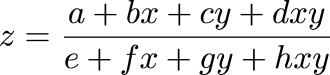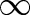Next Continued Fraction Arithmetic 38

# When to output?• Instead of evaluating one variable, x, on the range (0,)

• We evaluate two, x and y

• So we're asking what values z assumes on the entire quarter-plane

• Fortunately, it's enough to know its values only at the four corners [0, 0], [, 0], [0,], [,]

• These values are merely a/e, b/f, c/g, and d/h

• If all four agree on the next term, z can output it

• Otherwise, input from x or y

• Choose from x if |b/f - a/e| > |c/g - a/e|

 Next Back